Courses

# NCERT Solutions - The Theory Of The Firm Under Perfect Competition Commerce Notes | EduRev

## Commerce : NCERT Solutions - The Theory Of The Firm Under Perfect Competition Commerce Notes | EduRev

The document NCERT Solutions - The Theory Of The Firm Under Perfect Competition Commerce Notes | EduRev is a part of the Commerce Course Economics Class 11.
All you need of Commerce at this link: Commerce

Page No 66:

Question 1: What are the characteristics of a perfectly competitive market?
Perfect Competition
This type of market structure refers to the market that consists of a large number of buyers and also a large number of sellers. No individual seller is able to influence the price of an existing product in the market. All sellers in a perfect competition produce homogenous outputs, i.e. the outputs of all the sellers are similar to each other and the products are uniformly priced.
Features of Perfectly Competitive Market
1) A large number of buyers and sellers
There exist a large number of buyers and sellers in a perfectly competitive market. The number of sellers is so large that no individual firm owns the control over the market price of a commodity.
Due to the large number of sellers in the market, there exists a perfect and free competition. A firm acts as a price taker while the price is determined by the ‘invisible hands of market’, i.e. by ‘demand for’ and ‘supply of’ goods. Thus, we can conclude that under perfectly competitive market, an individual firm is a price taker and not a price maker.
2) Homogenous products
All the firms in a perfectly competitive market produce homogeneous products. This implies that the output of each firm is perfect substitute to others’ output in terms of quantity, quality, colour, size, features, etc. This indicates that the buyers are indifferent to the output of different firms. Due to the homogenous nature of products, existence of uniform price is guaranteed.
3) Free exit and entry of firms
In the long run there is free entry and exit of firms. However, in the short run some fixed factors obstruct the free entry and exit of firms. This ensures that all the firms in the long-run earn normal profit or zero economic profit that measures the opportunity cost of the firms either to continue production or to shut down. If there are abnormal profits, new firms will enter the market and if there are abnormal losses, a few existing firms will exit
the market.
4) Perfect knowledge among buyers and sellers
Both buyers and sellers are fully aware of the market conditions, such as price of a product at different places. The sellers are also aware of the prices at which the buyers are willing to buy the product. The implication of this feature is that if any individual firm is charging higher (or lower) price for a homogeneous product, the buyers will shift their purchase to other firms (or shift their purchase from the firm to other firms selling at lower price).
5) No transport costs
This feature means that all the firms have equal access to the market. The goods are produced and sold locally. Therefore, there is no cost of transporting the product from one part of the market to other.
6) Perfect mobility of factors of production
There exists geographically and occupationally perfect mobility of factors of production. This implies that the factors of production can move from one place to other and can move from one job to another.
7) No promotional and selling costs
There are no advertisements and promotional costs incurred by the firms. The selling costs under perfectly competitive market are zero.

Question 2: How are the total revenue of a firm, market price, and the quantity sold by that firm related to each other?
Answer: Total revenue is defined as the total sales proceeds of a producer by selling corresponding level of output. In other words, it is defined as price times the quantity of output sold.
Total Revenue = Price × Quantity of output sold
TR = P × Q
TR = PQ
In a perfectly competitive market, the market price is given, i.e., a firm acts as a price taker and cannot influence the price. Hence, a particular firm can influence its TR by altering the quantity of output sold.

Question 3: What is the price line?
Answer: Price line is the graphical representation of the relationship between output and price, with x-axis denoting the output and y-axis denoting the price. For a perfectly competitive firm, price line and demand curve are the same.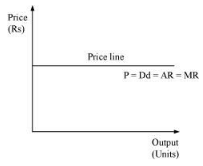Question 4: Why is the total revenue curve of a price-taking firm an upward-sloping straight line? Why does the curve pass through the origin?
Answer: The total revenue curve for a firm in a perfectly competitive market is an upward sloping curve because the price or AR remains constant and MR is also equal to AR. Thus, TR can only be influenced by altering the output sold, as the price remains constant. The increase in TR is in the same proportion as the increase in the output sold.
The curve passes through the origin, which implies that no matter what the price level is, if the output sold is zero, TR will also be zero.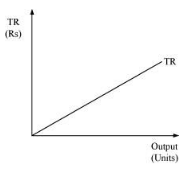Question 5: What is the relation between market price and average revenue of a price taking firm?
Answer: Average Revenue is defined as the revenue per unit of the output sold. It is expressed as the ratio between total revenue and the output sold.
AR=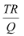We know that
TR = P × Q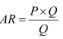AR = P
Thus, the market price and the average revenue are the same for a perfect competitive firm.

Question 6: What is the relation between market price and marginal revenue of a price-taking firm?
Answer: Marginal revenue is defined as the change in the total revenue that occurs due to the sale of one more unit of output. It is calculated as
MRn = TRn − TRn − 1
Where
MRn = Marginal revenue due to nth unit of output
TRn = Total revenue due to n units of output
TRn − 1 = Total revenue due to (n − 1) units of output
Suppose that the market price is P
MRn = TRn − TRn − 1
= PQn − P (Qn − 1)
MR = PQn − PQn+ P
MR = P
Thus, for a perfect competitive firm, marginal revenue is equal to the market price per unit of output.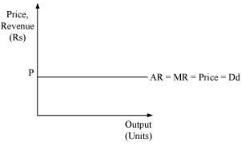Question 7: What conditions must hold if a profit-maximizing firm produces positive output in a competitive market?
Answer: The following three conditions must hold if a profit maximising firm produces positive level of output (say equilibrium output Q*) in a competitive market:
1) MR must be equal to MC at Q*.
2) MC should be upward sloping or rising at Q*.
3) In short run − Price must be greater than or equal to AVC. i.e. P ≥ AVC at Q*.
In long run − Price must be greater than or equal to LAC.

Question 8: Can there be a positive level of output that a profit-maximising firm produces in a competitive market at which market price is not equal to marginal cost? Give an explanation.
Answer: There cannot be any positive level of output that a firm produces at which price is not equal to MC. Let us evaluate the following two cases where price is not equal to MC.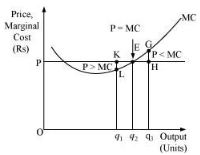Case A: If P > MC
At output Oq1, Price is Kq1, while the MC is Lq1. So, Oq1 is not the profit maximising output. This is due to the fact that the firm can increase its profit level by expanding its output to Oq2.
Case B: If P < MC
At output Oq3, price is Hq3 and MC is Gq3. So, Oq3 is not the profit maximising output. This is because the firm can increase its profit by reducing its output level to Oq2.
Thus, at profit maximising point, price must be equal to MC and it cannot be greater or lesser than MC.

Page No. 67

Question 9: Will a profit-maximising firm in a competitive market ever produce a positive level of output in the range where the marginal cost is falling? Give an explanation.
Answer: It is not possible for any perfect competitive firm to produce a positive level of output in a range where MC is falling. This is because, according to one of the conditions of profit-maximisation, MC curve should be upward sloping or the slope of MC curve should be positive at the equilibrium level of output.
Let us take an example:
At point Z price is equal to MC, but MC is falling and is negatively sloped. For any level of output more than Oq0, the firm is facing price > MC, which implies that the profit can be maximised by increasing the output level further.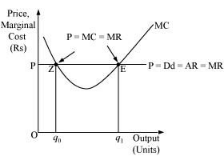Hence, the point ‘E’ is the equilibrium point, where a profit maximising firm would operate and produce Oq1 units of output and its profit will be maximised.

Question 10: Will a profit-maximising firm in a competitive market produce a positive level of output in the short run if the market price is less than the minimum of AVC?
It is not possible for a firm to produce positive level of output in the short run if the price is less than the minimum of AVC. This is because as soon as the market price falls below the minimum of SAVC, which implies that the firm is not able to cover its fixed as well as variable costs, and thus it will stop production.
Let us understand this concept by taking an example: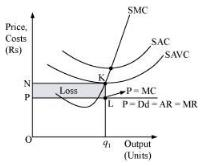At the point K, price charged by the firm is ON and output sold is Oq1, and the firm generates TR.
TR = P × Q
= OP × Oq1
= area (rectangle Oq1LP)
And incurs the variable cost of TVC
TVC = SAVC × Quantity of output
= ON × Oq1
= area (rectangle Oq1KN)
Profit earned by the firm = TR − TC = TR − (TVC + TFC)
= TR − TVC − TFC
If the firm is not producing anything then at zero level of output, the firm’s TR and TVC will be zero. However, the firm has to bear TFC. Thus at zero level of output, the profit earned by the firm is
Profit = π1 = TR − TVC − TFC
π1 = −TFC
Now if it produces Oq1 level of output, then the profit earned will be
π2 = TR − TVC − TFC
= area (rectangle Oq1LP) − area (rectangle Oq1KN) − TFC
Or, π2 = −area (rectangle PLKN) − TFC
This implies that π1 is greater than π2. The firm incurs more loss if it produces Oq1 level of output than the loss associated with zero level of output. Thus the firm will stop production whenever P < SAVC and therefore at profit maximising level of output, the price must be greater than or equal to SAVC in the short run.

Question 11: Will a profit-maximising firm in a competitive market produce a positive level of output in the long run if the market price is less than the minimum of AC? Give an explanation.
It is not possible for a firm to produce positive level of output in the long run if the market price falls short of the minimum of AC. This is because, in the long run there is free entry and exit of firms and all firms earn normal profit. Therefore, any firm making losses in long run will stop production.
Let us understand this concept through an example: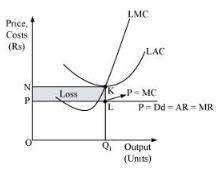At Oqlevel of output,
Price charged by the firm = OP.
Revenue generated by the firm (TR) = P × Q
= OP × Oq1
= area (rectangle Oq1LP)
Cost of producing Oq1 level of output (TC) = LAC × Quantity of output
= ON × Oq1
TC = area (rectangle Oq1KN)
Profit earned by the firm = TR − TC
= area (rectangle Oq1LP) − area (rectangle Oq1KN)
= − area (rectangle NKLP)
Thus, the loss incurred by the firm is equal to the area of the rectangle NKLP.
In the long run, all firms earn zero economic profit, and if any firm earns loss or negative profit, then the firm will shut down its production. Thus, if the firm earns loss, i.e. if price is lesser than LAC at any level of output, it will not be the profit maximising output level of the firm.

Question 12: What is the supply curve of a firm in the short run?
The short run supply curve of perfect competitive firm is the summation of the upward sloping portion of SMC (above the minimum point of SAVC), when price ≥ min SAVC, and vertical portion of price-axis, when price < min SAVC.
Stage 1
When the price is greater than or equal to minimum of SAVC, i.e., P ≥ min SAVC.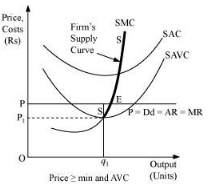At the market price OP, the three following conditions for equilibrium are fulfilled:
1.MC = MR
2.MC is upward sloping
3.Price exceeds the minimum of SAVC
At this market price the firm is producing profit maximising output Oq1.
In this case, the supply curve of the firm is regarded as the upward sloping part of SMC (above the minimum point of SAVC), i.e. SS. When the price is greater than or equal to minimum of SAVC, the supply curve is indicated by SS.
Stage 2
When the price is less than the minimum of SAVC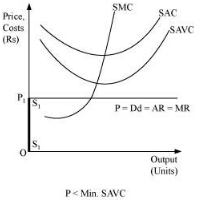Let us suppose that the firm is facing price OPthat is lesser than the minimum of SAVC. At this price, the firm cannot continue production as it cannot even cover up its variable costs and thereby incurs losses, which implies that the firm would produce nothing. Thus, it will incur loss that will be equivalent to its fixed costs. It will be lesser compared to the losses associated with producing any positive output level. Thus, the firm will not produce anything at this price and thereby the quantity supplied will be zero. The firm’s supply curve is indicated by the darkened vertical line S1S1.
Therefore, the short run supply curve of perfect competitive firm is (SS + S1S1).

Question 13: What is the supply curve of a firm in the long run?
Answer: In the long run, as there is no fixed cost, the perfectly competitive firm’s supply curve will be the summation of the upward sloping portion of SMC above the minimum point of LAC (when price ≥ minimum LAC), and the vertical portion of the price axis (when price < minimum of LAC). The long run supply curve of a perfect competitive firm is derived in two stages.
1. When price is equal to the minimum of LAC:
Let us suppose that the firm is facing market price OP that exceeds the minimum of LAC. MC is equal to MR (at point E) and MC is positively sloped at this point of intersection. Also, the price is greater than the minimum of LAC. Thus, the firm is at long run equilibrium, facing the price OP and producing Oq1 units of output. The supply curve is ‘SS’, represented by the upward portion of LMC above the minimum of LAC.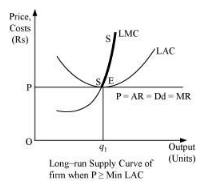2. When the price is less than the minimum of LAC:
Let us suppose that the market price faced by a firm is OP1, which is less than the minimum of LAC. At this price, the firm would not produce any output because producing any output will lead the firm to incur losses. Therefore, the firm would not produce anything. So, the supply curve of the firm in the long run for the price less than the minimum of LAC is given by S1S1 and is represented by the darkened vertical part of the price axis.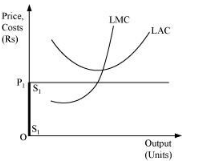Combining 1st and 2nd stages, the firm’s long run supply curve under perfect competition is given by (S1S1 + SS).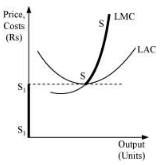Question 14: How does technological progress affect the supply curve of a firm?
Answer: The supply curve of a firm is a positive function of a state of technology. That is, if the technology available to the firm appreciates, more amount of output can be produced by the firm with the given levels of capital and labour. Due to such innovations or technological advancements, the firm will experience lower cost of production, which will lead to rightward downward shift of the MC curve. This will further lead to rightward shift of the firm’s supply curve. Thus, due to the appreciation and advancement of production techniques, the firm will produce more and more output that will be supplied at a given market price.

Question 15: How does the imposition of a unit tax affect the supply curve of a firm?
Answer: A unit tax is the tax imposed on per unit of the output sold. Due to the imposition of unit tax, the cost of production per unit of output increases, which ultimately increases the marginal cost. Consequently, the LMC curve will shift leftward upward and as the supply curve is a portion of LMC, so the supply curve will also shift leftward upward.
Let us understand the effect of imposition of unit tax through an example.
Suppose that a firm is facing the price OP1. LAC1 and LMC1 are the long run average cost curve and long run marginal cost curve respectively. Also assume that the government has imposed a unit tax of Rs k per unit of output produced. Now, this will rise the firm’s LAC and LMC, as the firm needs to pay Rs k extra on each output produced. Consequently, LMC1 and LAC1 will shift leftward upwards to LMC2 and LAC2. The magnitude of shift is equal to Rs k. As the supply curve is a part of LMC, it will also shift leftward from S1 to S2, due to the imposition of the tax. Consequently, the firm will now supply lesser units of output.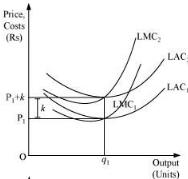Question 16: How does an increase in the price of an input affect the supply curve of a firm?
An increase in the price of an input increases the cost of production, which in turn increases the marginal cost of the firm. Consequently, the MC curve will shift upward to the left and the supply curve will also shift leftward upward. Therefore, an increase in the input price negatively affects the supply of the firm.

Question 17: How does an increase in the number of firms in a market affect the market supply curve?
The market supply curve is a horizontal summation of all the supply curves of individual firms in the market. If the number of firms in a market increases, then the market supply curve will shift rightward as there will be more number of firms supplying more amount of output.

Question 18: What does the price elasticity of supply mean? How do we measure it?
Price elasticity of supply (es) is defined as the degree of the responsiveness of quantity supplied, to the change in the price of a good.
It is expressed as: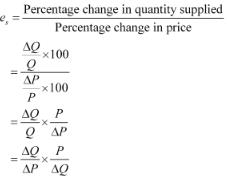Where
ΔQ = change in quantity supplied
ΔP = change in price
P = initial price
Q = initial supply

Question 19: Calculate the total revenue, marginal revenue and average revenue schedules in the following table. Market price of each unit of the good is Rs 10.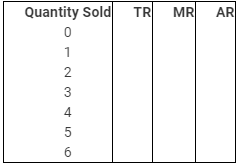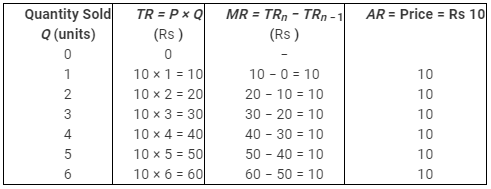Question 20: The following table shows the total revenue and total cost schedules of a competitive firm. Calculate the profit at each output level. Determine also the market price of the good.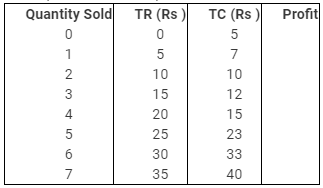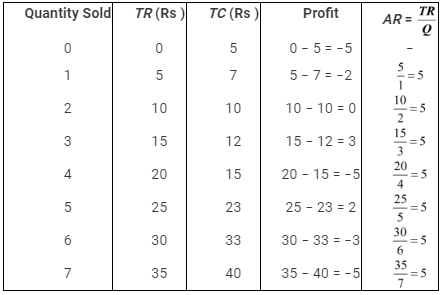Question 21: The following table shows the total cost schedule of a competitive firm. It is given that the price of the good is Rs 10. Calculate the profit at each output level. Find the profit maximising the level of output.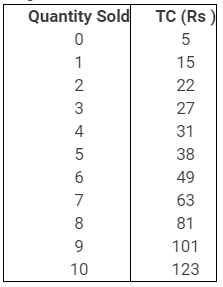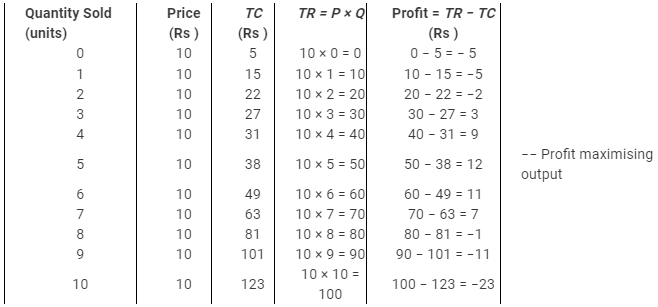Profit maximising output is where the difference between TR and TC is the maximum. This exists at 5 units of output, where firm is earning profit of Rs 1

Page No 68:

Question 22: Consider a market with two firms. The following table shows supply schedules of two firms: SS1 denotes the supply schedule of firm 1 and SS2 denotes the supply schedule of firm 2. Calculate the market supply schedule.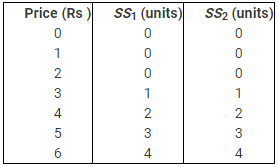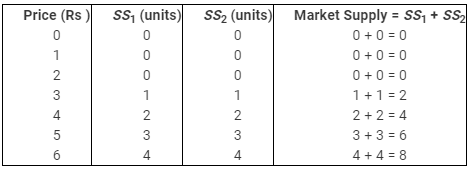Question 23: Consider a market with two firms. In the following table, columns labelled as SS1 and SS2 give the supply schedules of firm 1 and firm 2 respectively. Compute the market supply schedule.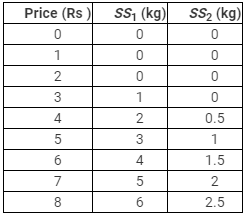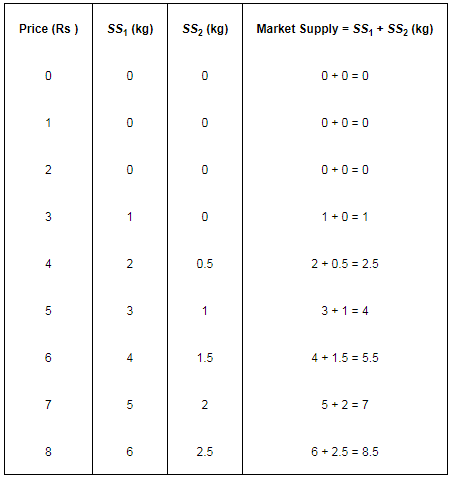Question 24: There are three identical firms in a market. The following table shows the supply schedule of firm 1. Calculate the market supply schedule.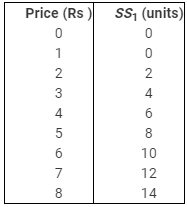As all the three firms are identical, so they have same individual supply curve and therefore the market supply will be the summation of supplies of all three firms.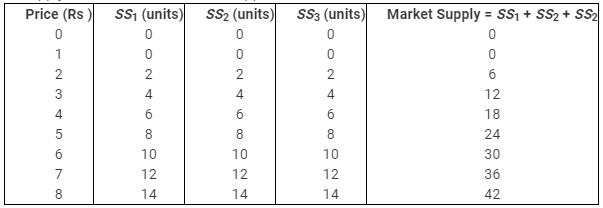Question 25: A firm earns a revenue of Rs 50 when the market price of a good is Rs 10. The market price increase to Rs 15 and the firm now earns a revenue of Rs 150. What is the price elasticity of the firm’s supply curve?
At Price, P1 = Rs 10
Total Revenue, TR1 = P1 × Q1 = 50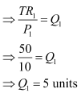At Price, P2 = Rs 15
Total Revenue, TR2 = P2 × Q2 = 150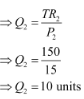Elasticity of supply,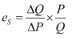ΔQ = Q2 − Q1 = 10 − 5 = 5
P = P1 − P2 = 15 − 10 = 5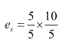es= 2

Question 26: The market price of a good changes from Rs 5 to Rs 20. As a result, the quantity supplied by a firm increases by 15 units. The price elasticity of the firm’s supply curve is 0.5. Find the initial and final output levels of the firm.
Answer: Elasticity of Supply, es = 0.5
Initial Price, P1 = Rs 5
Final price, P2 = Rs 20
ΔP = P2 − P1
= 20 − 5
ΔP = 15
ΔQ = 15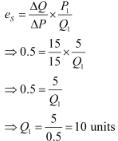Initial quantity = 10 units
Final quantity, Q2 = ΔQ + Q1
= 15 + 10
Therefore, Q2 = 25 units

Question 27: At the market price of Rs 10, a firm supplies 4 units of output. The market price increases to Rs 30. The price elasticity of the firm’s supply is 1.25. What quantity will the firm supply at the new price?

Initial Price, P1 = Rs 10
Initial Output, Q1 = 4 units
Final Price, P= Rs 30
ΔP = P2 − P1
= Rs 30 − 10 = Rs 20
Elasticity of supply, es = 1.25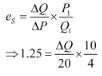⇒ 1.25 × 8 = ΔQ
⇒ ΔQ = 10 units
Thus final output supplied, Q2 = ΔQ + Q1
Q2 = 10 + 4 = 14 units

Offer running on EduRev: Apply code STAYHOME200 to get INR 200 off on our premium plan EduRev Infinity!

,

,

,

,

,

,

,

,

,

,

,

,

,

,

,

,

,

,

,

,

,

;